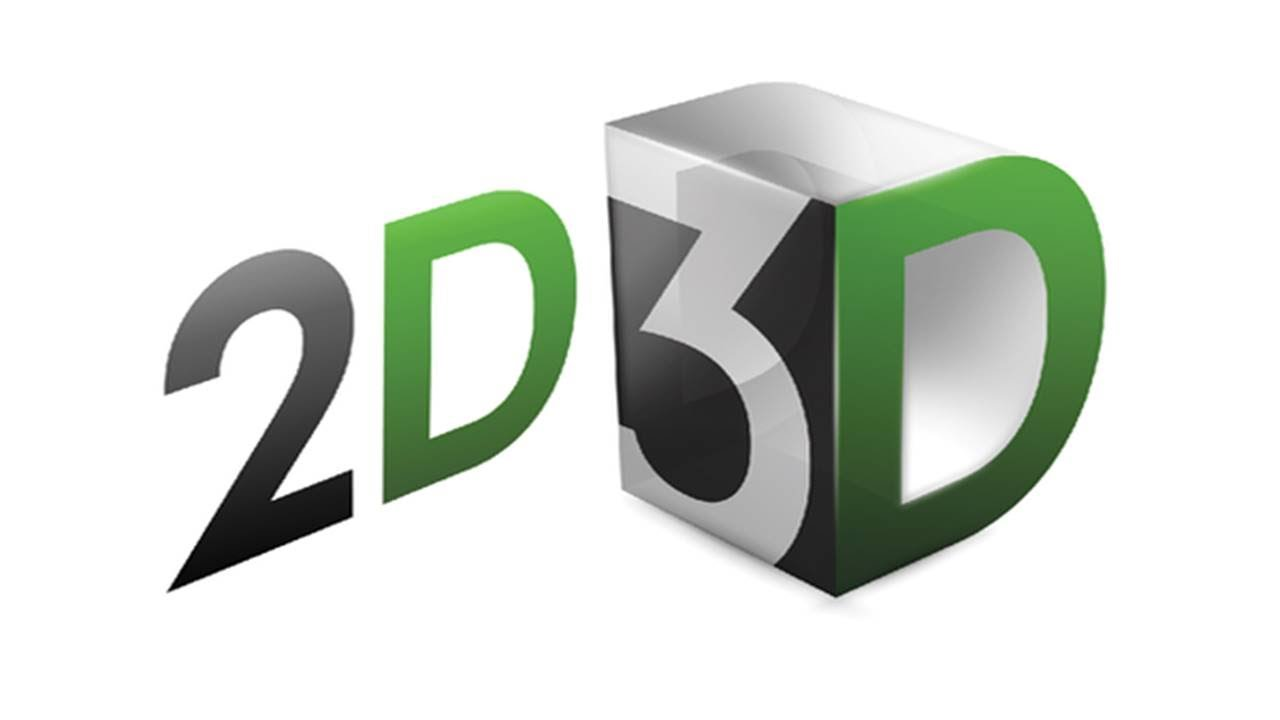Education

# What Are The Basic Geometrical Shapes In Maths?In Mathematics, a shape is an area closed by the boundary, formed by joining the number of dots, lines, and curves. Geometry is one of the main topics in mathematics. It is mainly utilized in configuring as well as the measurement of various shapes and sizes like circles, triangles, cubes, etc. It has wide usage in everyday life. Geometry is very essential in our lives to understand the different shapes plus measure them based on volumes & areas. Geometry is derived from the Greek world where Geo means Earth and metry means measurement. Therefore any object which presents on earth has a shape is defined by geometry.

There are different geometrical shapes in Mathematics. The geometrical shapes are basically classified into two different types, namely,

• Two Dimensional Shapes (2D shapes)
• Three Dimensional Shapes (3D shapes)

## Two-dimensional shapes

The two-dimensional shapes are also known as flat shapes or plane figures. It has only two dimensions, such as length and height, but it does not have a thickness. The examples of two-dimensional shapes are square, circle, triangle, rectangle, pentagon, hexagon, nonagon, and octagon. Each shape has different sides and angles. Apart from a circle, all the shapes are considered as a polygon. The two primary measures used to measure the flat shapes are Area and Perimeter. The area is the total space occupied by the figure. The perimeter is defined as the boundary of the object or the distance of the closed figure. The area of the 2D figure is usually measured in square units, whereas the perimeter is measured in units.

READ  Things to know before you join IAS Coaching institute in Delhi

Generally, in flat shapes, all the four-sided shapes are known as quadrilaterals. It includes a square, rectangle, rhombus, parallelogram, kite, and parallelogram. These quadrilaterals have more or less the same characteristic but differ from each by some distinct properties. For example, we can say that the area of the rhombus is equal to the area of the square if all the four vertices of the rhombus are at right angles. Thus, we can say that the square is a rhombus; however, the rhombus is not necessarily a square. The plane object or shape which has a length and breadth is called two-dimensional shapes. Generally, they have many numbers of sides.

## Three-dimensional shapes

The three-dimensional shapes are also known as solids. It has three different measures, such as length, breadth, and height. It means that 3D shapes have thickness. The solids are usually obtained from the 2D shapes. When rotation transformation is applied to the flat shapes, the solids are obtained. The 3D shapes are measured using the surface area and the volume. The volume is the total space occupied by the 3D shapes, whereas the surface area is the total area of the surface of solids. The surface areas of the solid shapes are usually two-dimensional shapes.

The area and the volume of any shape can be found using various approaches. For example, the area of an equilateral triangle is found using the basic triangle formula, rectangle construction, and trigonometry. Therefore any object which presents on earth has a shape is defined by geometry. Some of the three-dimensional shapes are a cube, sphere, cuboid, cylinder, ellipsoid, cone, pyramid, etc. In our daily life, we see numerous objects such as books, balls, etc with all various shapes. These objects have breadth, length, and height.